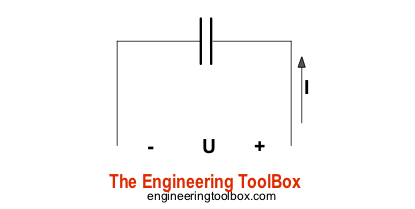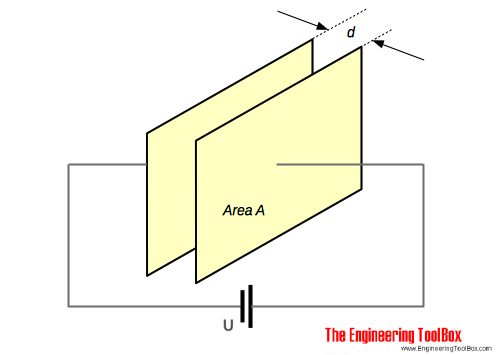Engineering ToolBox - Resources, Tools and Basic Information for Engineering and Design of Technical Applications!

# Capacitors

## Capacitors and capacitance - charge and unit of charge.

A capacitor is a device used to store electrical energy.The plates of a capacitor is charged and there is an electric field between them. The capacitor will be discharged if the plates are connected together through a resistor.

### Charge of a Capacitor

The charge of a capacitor can be expressed as

Q = I t                   (1)

where

Q = charge of capacitor (coulomb, C, mC)

I = current (amp, A)

t = time (s)

The quantity of charge (number of electrons) is measured in the unit Coulomb - C - where

1 coulomb = 6.24 1018 electrons

The smallest charge that exists is the charge carried by an electron, equal to -1.602 10-19 coulomb.

#### Example - Quantity of Electricity Transferred

If a current of 5 amp flows for 2 minutes, the quantity of electricity - coulombs - can be calculated as

Q = (5 A) (2 min) (60 s/min)

= 600

or, in electrons:

(600 C) (6.24 1018 electrons / C)

= 3.744 1021 electrons

### Electric Field Strength (Dielectric Strength)If two charged plates are separated with an insulating medium - a dielectric -  the electric field strength (potential gradient) between the two plates can be expressed as

E = U / d                        (2)

where

E = electric field strength (volts/m)

U = eletrical potential (volt)

d = thickness of dielectric, distance between plates (m)

#### Example - Electric Field Strength

The voltage between two plates is 230 V and the distance between them is 5 mm. The electric field strength can be calculated as

E = (230 V) / ((5 mm) (10-3 m/mm))

= 46000 volts/m

= 46 kV/m

### Electric Flux Density

Electric flux density is the ratio between the charge of the capacitor and the surface area of the capacitor plates:

D = Q / A                       (3)

where

D = electric flux density (coulomb/m2)

A = surface area of the capacitor (m2)

### Charge and Applied Voltage

Charge in a capacitor is proportional to the applied voltage and can be expressed as

Q = C U                        (4)

where

C = constant of proportionality or capacitance (farad, F, µF )

### Capacitance

From (4) the capacitance can be expressed as

C = Q / U                         (5)

One farad is defined as the capacitance of a capacitor when there is a potential difference across the plates of one volt when holding a charge of one coulomb.

It is common to use µF (10-6 F)

#### Example - Voltage over a Capacitor

A 5 µF capacitor is charged with 10 mC. The voltage across the capacitor can be calculated by modifying (4) to

U = Q / C

= (10 mC) (10-3 C/mC) / ((5 µF) (10-6 F/µF)

= 2000 V

= 2 kV

### Absolute Permittivity

The ratio of electric flux density to electric field is called absolute permittivity - ε - of a dielectric and can be expressed as

ε = D / E                     (6)

where

ε = absolute permittivity (F/m)

The absolute permittivity of free space or vacuum - also called the electric constant - ε0 - is 8.85 10-12 F/m.

### Relative Permittivity

Relative permittivity - also called the dielectric constant  εr - is the ratio between the flux density of the field in an actual dielectric - ε - and the flux density of the field in absolute vacuum - ε0.

εr = ε / ε0                   (7a)

The actual permittivity can be calculated by transforming (7a) to

ε = εr ε0                      (7b)

### Parallel Plate Capacitor

The capacitance of a plate capacitor - as shown in the figure above - is proportional with the area A of the plate. The capacitance can be expressed as

C = εr ε0 A / d                   (8)

where

A = area of plate (m2)

d = thickness of dielectric, distance between plates (m)

For a plate capacitor with multiple plates the capacitance can be expressed as

C = εr ε0 A (n - 1) / d                      (8b)

where

n = number of plates

### Example - Capacitance of a Plate Capacitor

The capacitance of a plate capacitor with area 5 cm2, 10 plates and distance 0.1 mm between the plates - with ceramic dielectric with relative permittivity 30 between the plates - can be calculated as

C = (8.85 10-12 F/m) (30) (5 cm2) (10-4 m2/cm2) (10 - 1) / ((0.1 mm) (10-3 m/mm))

= 11 10-9 F

= 11 pF

### Typical commonly used Capacitors

Typical capacitors are

• variable air capacitors
• mica capacitors
• paper capacitors
• ceramic capacitors
• plastic capacitors
• titanium oxide capacitors
• electrolytic capacitors

## Related Topics

• ### Electrical

Electrical units, amps and electrical wiring, wire gauge and AWG, electrical formulas and motors.

## Related Documents

• ### Acceleration

Change in velocity vs. time used.
• ### Capacitors - Parallel and Serial Connections

Parallel and serial connected capacitor circuits.
• ### Capacitors - Stored Energy

Potential power and energy stored in capacitors.
• ### Electrical Conductivity - Elements and other Materials

Electric conductance is the ability of a element to conduct an electric current.
• ### Electromotive Force - e.m.f

Change in electrical potential between two points.
• ### Relative Permittivity - the Dielectric Constant

Common materials and their relative permittivity.

## Engineering ToolBox - SketchUp Extension - Online 3D modeling!

Add standard and customized parametric components - like flange beams, lumbers, piping, stairs and more - to your Sketchup model with the Engineering ToolBox - SketchUp Extension - enabled for use with older versions of the amazing SketchUp Make and the newer "up to date" SketchUp Pro . Add the Engineering ToolBox extension to your SketchUp Make/Pro from the Extension Warehouse !

We don't collect information from our users. More about

## Citation

• The Engineering ToolBox (2008). Capacitors. [online] Available at: https://www.engineeringtoolbox.com/capacitors-d_1387.html [Accessed Day Month Year].

Modify the access date according your visit.

9.29.12